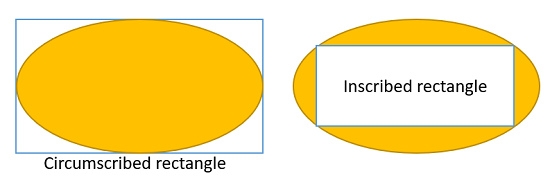# Inscribed Rectangle & Circumscribed RectangleAn inscribed rectangle is a rectangle drawn within a shape. In calculus, we’re mostly concerned with the largest inscribed rectangle; The largest that doesn’t break through the edges of the shape. A circumscribed rectangle is drawn outside of a shape; Usually we want to know the smallest rectangle that can completely enclose a shape.

## Use of Inscribed and Circumscribed Rectangles in Calculus

Inscribed rectangles and circumscribed rectangles are used in a few different areas in calculus, most notably in Riemann Sums and Optimization.

## Inscribed rectangles and circumscribed rectangles in Riemann Sums

The area under a curve can be approximated with a series of rectangles:

• Inscribed rectangles are an under-approximation of the area (called a lower sum).
• Circumscribed rectangles are an over-approximation of the area (called an upper sum).

For step by step examples and how-to video, see: Riemann sums.

## 2. Optimization: Largest Inscribed Rectangle Within a Circle

Many optimization problems (which deal with the “biggest” or “smallest”) use inscribed and circumscribed rectangles.

Example: What is the largest inscribed rectangle that can fit into a circle with a radius of 1?Step 1: Formulate a function to maximize.

We know that the diagonal of any inscribed rectangle (blue line) has a length of 2 (because 2 * radius = diameter). We can use this information and the Pythagorean theorem (a2 + b2 = c2) to get:

w2 + h2 = 4.

We need to get this formula in terms of one variable to create our function:

1. Solve for w: w2 + h2 = 4 → w = √(4 – h)2.
2. Substitute (1) into A = w * h → A = h * √(4 – h2).

The formula we need to maximize is A = h * √(4 – h2).

Step 2: Graph the function (I used Desmos.com). We want to find the function’s maximum height.The function has a maximum x-value (actually, our “h”) at x = √2 ≈ 1.414. Therefore, the largest rectangle has a height of √2.

If h = √2, then, putting that into the Pythagorean theorem:
1.4142 + b2 = 4
2 + b2 = 4
2 + √2,2 = 4
2 + 2 = 4

The inscribed rectangle is a 2 x 2 square with an area of 4.

CITE THIS AS:
Stephanie Glen. "Inscribed Rectangle & Circumscribed Rectangle" From StatisticsHowTo.com: Elementary Statistics for the rest of us! https://www.statisticshowto.com/inscribed-rectangle-circumscribed/